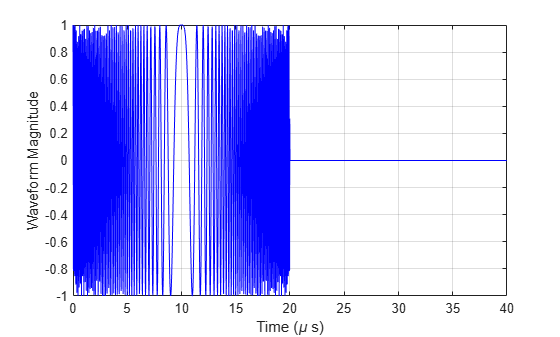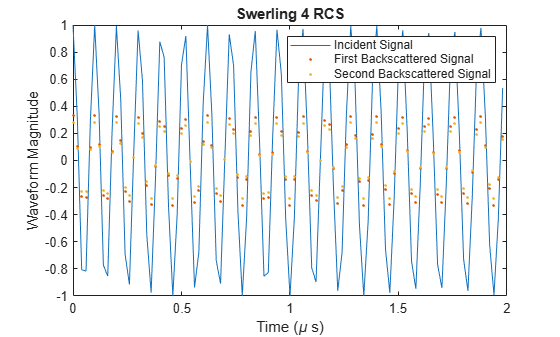# step

Package: phased

Backscatter wideband signal from radar target

## Syntax

refl_sig = step(target,sig,ang)
refl_sig = step(target,sig,ang,update)
refl_sig = step(target,sig,ang,laxes)
refl_sig = step(target,sig,ang,laxes,update)

## Description

Note

Alternatively, instead of using the step method to perform the operation defined by the System object™, you can call the object with arguments, as if it were a function. For example, y = step(obj,x) and y = obj(x) perform equivalent operations.

example

refl_sig = step(target,sig,ang) returns the reflected signal, refl_sig, of an incident nonpolarized signal, sig. This syntax applies when you set the EnablePolarization property to false and the Model property to 'Nonfluctuating'. In this case, the values specified in the RCSPattern property are used to compute the RCS values for the incident and reflected directions, ang.

example

refl_sig = step(target,sig,ang,update) uses update to control whether to update the RCS values. This syntax applies when you set the EnablePolarization property to false and the Model property to one of the fluctuating RCS models: 'Swerling1', 'Swerling2', 'Swerling3', or 'Swerling4'. If update is true, a new RCS value is generated. If update is false, the previous RCS value is used.
 refl_sig = step(target,sig,ang,laxes) returns the reflected signal, refl_sig, of an incident polarized signal, sig. This syntax applies when you set EnablePolarization to true and the Model property to 'Nonfluctuating'. The values specified in the ShhPattern, SvvPattern, and ShvPattern properties are used to compute the backscattering matrices for the incident directions, ang. The laxes argument specifies a local coordinate system used to define the horizontal and vertical polarization components.
 refl_sig = step(target,sig,ang,laxes,update) uses the update argument to control whether to update the scattering matrix values. This syntax applies when you set the EnablePolarization property to true and the Model property to one of the fluctuating RCS models: 'Swerling1', 'Swerling2', 'Swerling3', or 'Swerling4'. If update is true, a new RCS value is generated. If update is false, the previous RCS value is used.

Note

The object performs an initialization the first time the object is executed. This initialization locks nontunable properties and input specifications, such as dimensions, complexity, and data type of the input data. If you change a nontunable property or an input specification, the System object issues an error. To change nontunable properties or inputs, you must first call the release method to unlock the object.

## Input Arguments

expand all

Backscatter target, specified as a phased.WidebandBackscatterRadarTarget System object.

• Wideband nonpolarized signal, specified as an N-by-M complex-valued matrix. The quantity N is the number of signal samples and M is the number of independent signals reflecting off the target. Each column contains an independent signal reflected from the target.

The size of the first dimension of the input matrix can vary to simulate a changing signal length. A size change can occur, for example, in the case of a pulse waveform with variable pulse repetition frequency.

• Wideband polarized signal, specified as a 1-by-M struct array containing complex-valued fields. Each struct element contains three N-by-1 column vectors of electromagnetic field components (sig.X,sig.Y,sig.Z) representing the polarized signal that reflects from the target. Each struct element contains three N-by-1 complex-valued column vectors, sig.X, sig.Y, and sig.Z. These vectors represent the x, y, and z Cartesian components of the polarized signal.

The size of the first dimension of the matrix fields within the struct can vary to simulate a changing signal length such as a pulse waveform with variable pulse repetition frequency.

Example: [1,1;j,1;0.5,0]

Data Types: double
Complex Number Support: Yes

Incident signal direction, specified as a real-valued column 2-by-1 vector or 2-by-M matrix of positive values. Each column of ang specifies the incident direction of the corresponding signal in the form [AzimuthAngle;ElevationAngle]. The number of columns in ang must match the number of independent signals in sig. Units are in degrees.

Example: [30;45]

Data Types: double

Option to enable the RCS values for fluctuation models to update, specified as false or true. When update is true, a new RCS value is generated with each call to the step method. If update is false, the RCS remains unchanged with each call to step.

Data Types: logical

Local coordinate system matrix, specified as a 3-by-3 real-valued orthonormal matrix or a 3-by-3-by-M real-valued array. The matrix columns specify the local coordinate system orthonormal x-axis, y-axis, and z-axis, respectively. Each axis is a vector of the form (x;y;z) with respect to the global coordinate system. When sig has only one signal, laxes is a 3-by-3 matrix. When sig has multiple signals, you can use a single 3-by-3 matrix for multiple signals in sig. In this case, all targets have the same local coordinate systems. When you specify laxes as a 3-by-3-by-M array, each page (third index) defines a 3-by-3 local coordinate matrix for the corresponding target.

Example: [1,0,0;0,0.7071,-0.7071;0,0.7071,0.7071]

Data Types: double

## Output Arguments

expand all

• Wideband nonpolarized signal, returned as an N-by-M complex-valued matrix. Each column contains an independent signal reflected from the target.

• Wideband polarized signal, returned as a 1-by-M struct array containing complex-valued fields. Each struct element contains three N-by-1 column vectors of electromagnetic field components (sig.X,sig.Y,sig.Z) representing the polarized signal that reflects from the target.

The quantity N is the number of signal samples and M is the number of signals reflecting off the target. Each column corresponds to a reflecting angle.

For polarized fields, the struct element contains three N-by-1 complex-valued column vectors: sig.X, sig.Y, and sig.Z. These vectors represent the x, y, and z Cartesian components of the polarized signal.

The output refl_sig contains signal samples arriving at the signal destination within the current input time frame. When the propagation time from source to destination exceeds the current time frame duration, the output does not contain all contributions from the input of the current time frame. The remaining output appears in the next call to step.

## Examples

expand all

Calculate the reflected radar signal from a nonfluctuating point target having a peak RCS of 10.0 m^2. Use a simple target RCS pattern for illustrative purposes. Real RCS patterns are more complicated. The RCS pattern covers a range of angles from $10-30$ degrees in azimuth and $5-15$ degrees in elevation. The RCS peaks at 20 degrees azimuth and 10 degrees elevation. The RCS also has a frequency dependence and is specified at 5 frequencies within the signal bandwidth. Assume that the radar operating frequency is 100 MHz and that the signal is a linear FM waveform having a 20 MHz bandwidth.

Create and plot the wideband signal.

c = physconst('LightSpeed'); fs = 50e6; pw = 20e-6; PRF = 1/(2*pw); fc = 100e6; bw = 20e6; waveform = phased.LinearFMWaveform('SampleRate',fs,'PulseWidth',pw, ... 'PRF',PRF,'OutputFormat','Pulses','NumPulses',1,'SweepBandwidth',bw, ... 'SweepDirection','Down','Envelope','Rectangular','SweepInterval', ... 'Symmetric'); wav = waveform(); n = size(wav,1); plot([0:(n-1)]/fs*1e6,real(wav),'b') xlabel('Time (\mu s)') ylabel('Waveform Magnitude') gridCreate an RCS pattern at five different frequencies within the signal bandwidth using a simplified frequency dependence. The frequency dependence is unity at the operating frequency and falls off outside that frequency. Realistic frequency dependencies are more complicated. Plot the RCS pattern for one of the frequencies.

fvec = fc + [-fs/2,-fs/4,0,fs/4,fs/2]; fdep = cos(3*(1 - fvec/fc)); azmax = 20.0; elmax = 10.0; azpattern = [10.0:0.5:30.0]; elpattern = [5.0:0.5:15.0]; rcspattern0 = 10.0*cosd(4*(elpattern - elmax))'*cosd(4*(azpattern - azmax)); for k = 1:5 rcspattern(:,:,k) = rcspattern0*fdep(k); end imagesc(azpattern,elpattern,abs(rcspattern(:,:,1))) axis image axis tight title('RCS') xlabel('Azimuth (deg)') ylabel('Elevation (deg)')Create the phased.WidebandBackscatterRadarTarget System object™.

target = phased.WidebandBackscatterRadarTarget('Model','Nonfluctuating', ... 'AzimuthAngles',azpattern,'ElevationAngles',elpattern,... 'RCSPattern',rcspattern,'OperatingFrequency',fc,'NumSubbands',32, ... 'FrequencyVector',fvec);

For a sequence of incident azimuth angles at constant elevation, find and plot the reflected signal amplitude.

az0 = 13.0; el = 10.0; az = az0 + [0:2:20]; naz = length(az); magsig = zeros(1,naz); for k = 1:naz y = target(wav,[az(k);el]); magsig(k) = max(abs(y)); end plot(az,magsig,'r.') xlabel('Azimuth (deg)') ylabel('Scattered Signal Amplitude') gridCalculate the reflected radar signal from a Swerling 4 fluctuating point target with a peak RCS of 0.1 m^2. Use a simple target RCS pattern for illustrative purposes. Real RCS patterns are more complicated. The RCS pattern covers a range of angles from 10 $-$ 30 degrees in azimuth and 5 $-$15 degrees in elevation. The RCS peaks at 20 degrees in azimuth and 10 degrees in elevation at a value of 0.1 m^2. The RCS also has a frequency dependence and is specified at five frequencies within the signal bandwidth. Assume that the radar operating frequency is 100 MHz and that the signal is a linear FM waveform with a 20 MHz bandwidth. The sampling frequency is 50 MHz.

Create and plot the wideband signal.

c = physconst('LightSpeed'); fs = 50e6; pw = 20e-6; PRF = 1/(2*pw); fc = 100.0e6; bw = 20.0e6; waveform = phased.LinearFMWaveform('SampleRate',fs,'PulseWidth',pw, ... 'PRF',PRF,'OutputFormat','Pulses','NumPulses',1,'SweepBandwidth',bw, ... 'SweepDirection','Down','Envelope','Rectangular','SweepInterval', ... 'Symmetric'); wav = waveform();

Create an RCS pattern at five different frequencies within the signal bandwidth using a simple frequency dependence. The frequency dependence is designed to be unity at the operating frequency and fall off outside that band. Realistic frequency dependencies are more complicated.

fvec = fc + [-fs/2,-fs/4,0,fs/4,fs/2]; fdep = cos(3*(1 - fvec/fc)); azmax = 20.0; elmax = 10.0; azangs = [10.0:0.5:30.0]; elangs = [5.0:0.5:15.0]; rcspattern0 = 0.1*(cosd((elangs - elmax))'*cosd((azangs - azmax))).^2; for k = 1:5 rcspattern(:,:,k) = rcspattern0*fdep(k); end imagesc(azangs,elangs,abs(rcspattern(:,:,5))) axis image axis xy axis tight title('RCS') xlabel('Azimuth (deg)') ylabel('Elevation (deg)') colorbarCreate the phased.WidebandBackscatterRadarTarget System object™.

target = phased.WidebandBackscatterRadarTarget('Model','Swerling4', ... 'SeedSource','Property','Seed',100213,'AzimuthAngles',azangs, ... 'ElevationAngles',elangs,'RCSPattern',rcspattern, ... 'OperatingFrequency',fc,'NumSubbands',32,'FrequencyVector',fvec);

Find and plot 100 samples of the incident signal and two sequential reflected signals at 10 degrees in azimuth and 10 degrees in elevation. Update the RCS at each execution of the System object™.

az = 10.0; el = 10.0; refl_wav1 = target(wav,[az;el],true); refl_wav2 = target(wav,[az;el],true); n = 100; plot([0:(n-1)]/fs*1e6,real(wav(1:n))) hold on plot([0:(n-1)]/fs*1e6,real(refl_wav1(1:n)),'.') plot([0:(n-1)]/fs*1e6,real(refl_wav2(1:n)),'.') hold off legend('Incident Signal','First Backscattered Signal','Second Backscattered Signal') xlabel('Time (\mu s)') ylabel('Waveform Magnitude') title('Swerling 4 RCS')## Version History

Introduced in R2016b